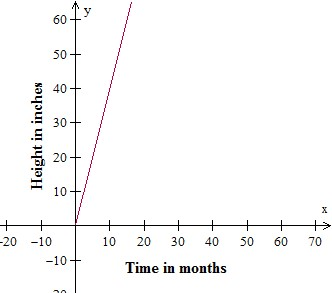# Identifying independent and dependent quantities from tables and graphs Online Quiz

#### Excel Pivot Tables and Dashboard. Simple & Quick tutorial!

Most Popular

7 Lectures 58 mins

#### Learn HTML Tables

15 Lectures 59 mins

#### Excel Analytics - Data Analysis with Pivot-Tables and Charts

46 Lectures 2.5 hours

Following quiz provides Multiple Choice Questions (MCQs) related to Identifying independent and dependent quantities from tables and graphs. You will have to read all the given answers and click over the correct answer. If you are not sure about the answer then you can check the answer using Show Answer button. You can use Next Quiz button to check new set of questions in the quiz.### Explanation

Independent variable is Distance traveled in miles; Dependent variable is Bus fare in dollars.

Q 2 - Identify the dependent and independent variables in the following problem.### Explanation

Independent variable is Distance in miles; Dependent variable is Cab fare in dollars.

Q 3 - Identify the dependent and independent variables in the following problem.

A freight truck moves at a constant speed. The distance y in miles that the truck has traveled after x hours is shown in the table.

 Time in hours (x) Distance in miles (y) 0 1 2 3 0 60 120 180

### Explanation

Independent variable is Time in hours; Dependent variable is Distance in miles.

### Explanation

Independent variable is Clay bought by teacher; Dependent variable is Clay available for class

Q 5 - Identify the dependent and independent variables in the following problem.

Time in years versus the rent in dollars is shown in the table below.

 Time in years (x) Rent in dollars (y) 1 2 3 4 2000 4000 6000 8000

### Explanation

Independent variable is Time in years; Dependent variable is Rent in dollars.

Q 6 - Identify the dependent and independent variables in the following problem.### Explanation

Independent variable is Number of pages; Dependent variable is Time in minutes.

Q 7 - Identify the dependent and independent variables in the following problem.

Time in years versus the weight in pounds is shown in the table below.

 Time in years (x) Weight in pounds (y) 0 1 2 3 0 50 100 150

### Explanation

Independent variable is Time in years; Dependent variable is Weight in pounds.

Q 8 - Identify the dependent and independent variables in the following problem.### Explanation

Independent variable is Number in minutes; Dependent variable is Phone bill in dollars.

Q 9 - Identify the dependent and independent variables in the following problem.

The number of pages read versus the time taken in minutes is shown in the table below.

 Number of pages (y) Time in minutes (x) 2 4 6 8 4 8 12 16

### Explanation

Independent variable is Number of pages read; Dependent variable is Time in minutes.

Q 10 - Identify the dependent and independent variables in the following problem.### Explanation

Independent variable is Time in months; Dependent variable is Height in inches.

identifying_independent_and_dependent_quantities_from_tables_and_graphs.htm